Get instant live expert help with Excel or Google Sheets“My Excelchat expert helped me in less than 20 minutes, saving me what would have been 5 hours of work!”

#### Post your problem and you’ll get Expert help in seconds.

Your message must be at least 40 characters
Our professional Expert are available now. Your privacy is guaranteed.

# Excel ARABIC FunctionRead time: 15 minutes

While working with Excel, we are able to convert a Roman numeral into its Arabic numeral form by using the ARABIC function.  This step by step tutorial will assist all levels of Excel users in the syntax and usage of ARABIC function.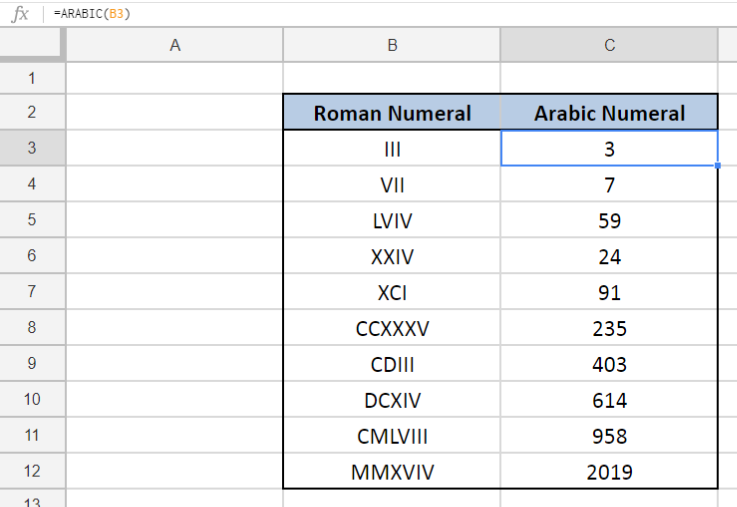Figure 1. Final result: Excel ARABIC function

## Syntax of ARABIC Function

ARABIC returns the value of a Roman numeral; converts a Roman numeral into an Arabic numeral

`=ARABIC(roman_numeral)`

• roman_numeral – the Roman numeral we want to convert to an Arabic number; can be a string enclosed in quotation marks, or a cell reference
• ARABIC function performs the opposite of the ROMAN function

Below table shows the Roman numeral symbols and their corresponding values.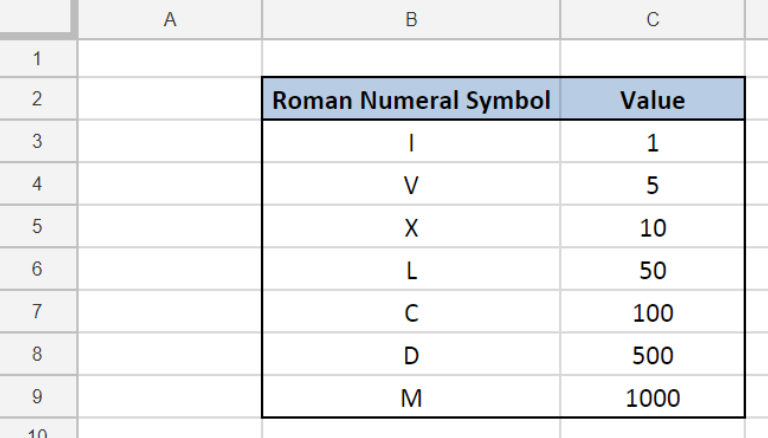Figure 2. Roman numeral symbols and values

## Setting up our Data

Our data consists of two columns: Roman Numeral (column B) and Arabic Numeral (column C).  We want to convert the Roman numeral into its Arabic numeral form. The results will be recorded in column C.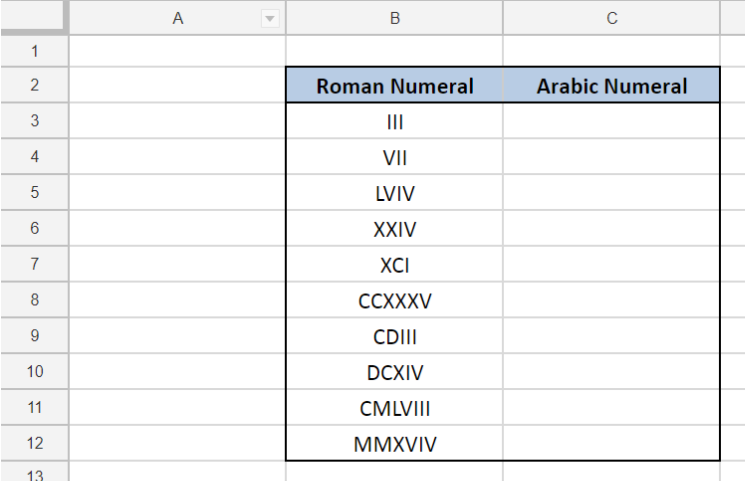Figure 3. Sample data to convert to Arabic numeral

## Convert to Arabic numeral

In order to convert a Roman numeral into an Arabic numeral, let us follow these steps:

Step 1.  Select cell C3

Step 2.  Enter the formula: `=ARABIC(B3)`

Step 3.  Press ENTER

Step 4:  Copy the formula in cell C3 to cells C4:C12 by clicking the “+” icon at the bottom-right corner of cell C3 and dragging it down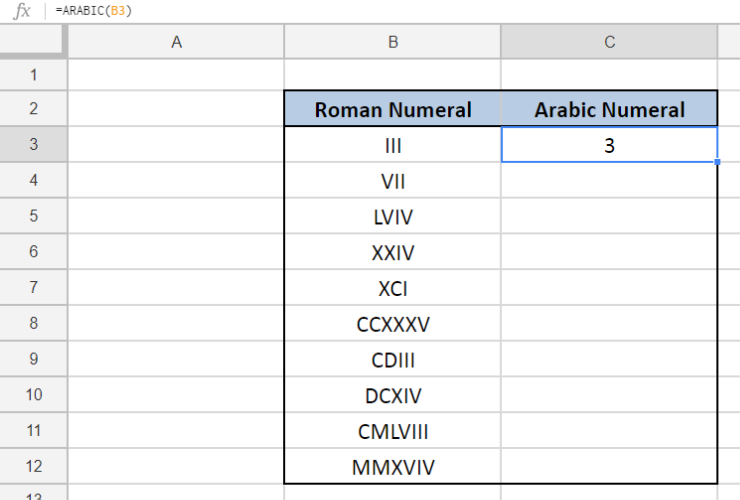Figure 4.  Using the ARABIC function to convert from Roman to Arabic numeral

The corresponding Arabic numeral value of B3 III is “3”, as shown in cell C3.

For the succeeding examples, the Roman numerals are converted to their corresponding Arabic numeral forms in cells C4 to C12.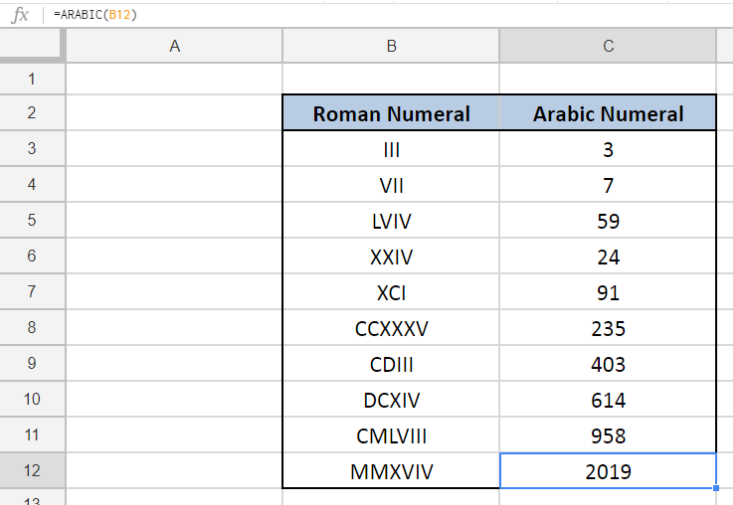Figure 5.  Output : Roman Numeral converted to ARABIC numeral

The ARABIC function can be used to convert any Roman numeral, as long as the argument is a valid representation of a Roman numeral.

## Notes:

• ARABIC function is not case-sensitive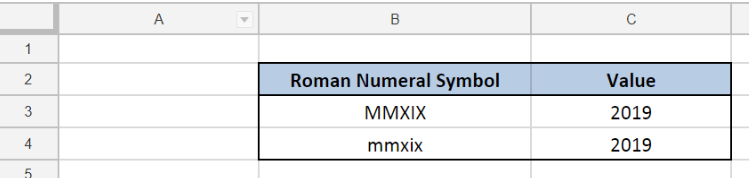Figure 6.  ARABIC function is not case-sensitive

• If the input value is an empty string “”, ARABIC function returns a zero “0”
• ARABIC returns the error #VALUE! when the argument for Roman numeral is invalid; Examples of invalid input values are:
• Dates
• Numbers
• Characters that are not symbols for Roman numerals (e.g. “A”)
• Values with space in between the symbols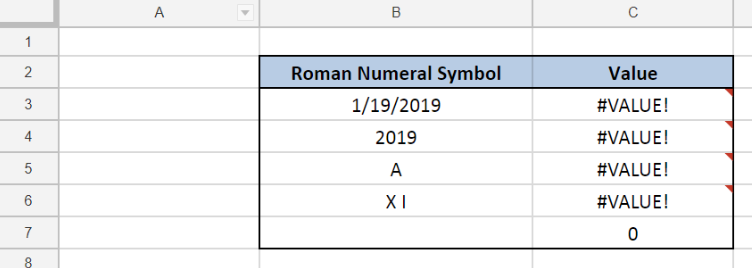Figure 7.  Examples of invalid input values for ARABIC function

Most of the time, the problem you will need to solve will be more complex than a simple application of a formula or function. If you want to save hours of research and frustration, try our live Excelchat service! Our Excel Experts are available 24/7 to answer any Excel question you may have. We guarantee a connection within 30 seconds and a customized solution within 20 minutes.

### Did this post not answer your question? Get a solution from connecting with the expert.Another blog reader asked this question today on Excelchat:
Solution examplesI need a formula that has these 2 rules. If letter was rec'd between 1st and 15th = 1st of the month following letter rec'd date. If letter was rec'd between 16thst and end of the month= 1st of the 2nd month following letter rec'd date.
Solved by V. F. in 20 minsI need a column to total (Hours)(85) + (Travel)(.55) per line
Solved by F. Q. in 20 minsTrying to figure out what is wrong with my formula in Oct-ZD tab in column AY and BG.
Solved by O. Q. in 8 minsSo I have used vlookup to find the price of a product on a different sheet and then I have tried to times that by a quantity. e.g. =vlookup(d4,table35,2,false)*e4 This is the formula I typed in which worked for another list I did but for some reason it's not working now even though everything is practically the same, apart from the contents. Could you help?
Solved by B. U. in 60 minsI have a column containing date and time data together and i need to isolate the time data. When I tried to use text to column, it changed all the times to AM. There is an extremely large amount of data - over 550,000 rows so manually changing it won't work. The file is too large to attach.
Solved by S. E. in 40 mins## Subscribe to Excelchat.coAnother blog reader asked this question today on Excelchat: